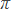Standard:

Description:

Standard:

# Math.8.NS.2 or 8.NS.A.2

Description:

## Use rational approximations of irrational numbers to compare the size of irrational numbers, locate them approximately on a number line diagram, and estimate the value of expressions (e.g.,2). For example, by truncating the decimal expansion of √2, show that √2 is between 1 and 2, then between 1.4 and 1.5, and explain how to continue on to get better approximations.8th Grade Math - Real Numbers Lesson